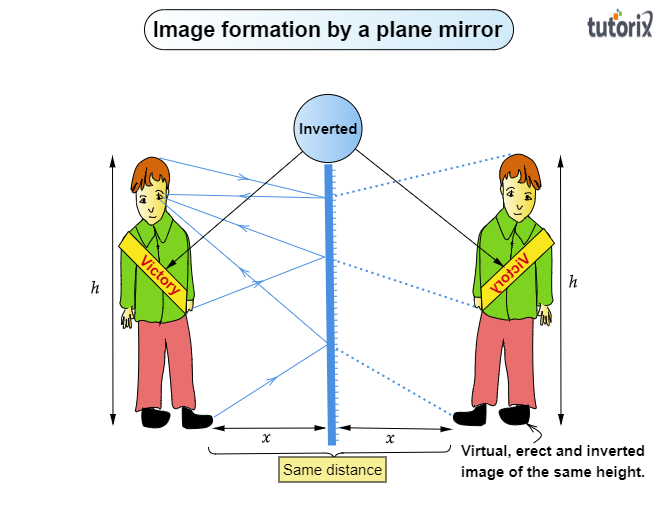# State the characteristics of the image formed by a plane mirror.

Characteristics of the image of the objects formed by a plane mirror:

1. The image formed by a plane mirror is virtual (behind the mirror)

2. The image formed by a plane mirror is erect (upright).

3. The size of the image formed by a plane mirror is the same as that of the size of the object.

For example- If an object is 15 cm high, then the image of this object will also be 15 cm high.

4. The distance of the image formed by the plane mirror, is equal to the distance of the object from the mirror.

For example- Suppose, an object is placed at 10 cm in front of a plane mirror then its image will be at 10 cm behind the plane mirror.

Ray diagram of image formation by a plane mirror:

In the ray diagram below, the image formed by a plane mirror is virtual (behind the mirror), erect (upright), laterally inverted, same as that of the size of the object, and is equal to the distance of the object from the mirror.